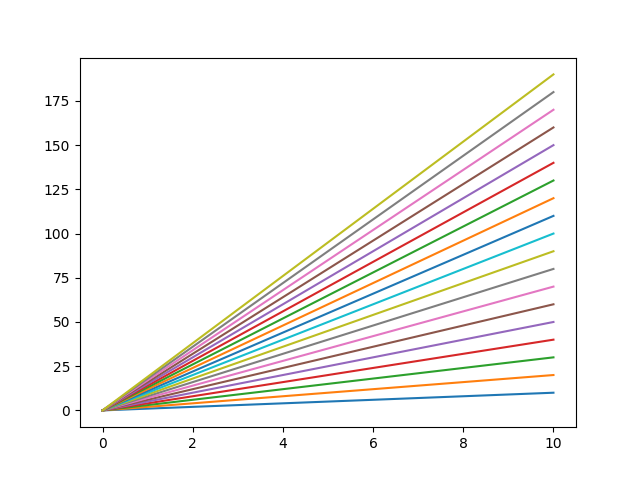# Highlighting the artist upon selection¶

Just pass `highlight=True` to `cursor`.```import numpy as np
import matplotlib.pyplot as plt
import mplcursors

x = np.linspace(0, 10, 100)

fig, ax = plt.subplots()

# Plot a series of lines with increasing slopes.
lines = []
for i in range(1, 20):
line, = ax.plot(x, i * x, label=f"\$y = {i}x\$")
lines.append(line)

mplcursors.cursor(lines, highlight=True)

plt.show()
```

Gallery generated by Sphinx-Gallery